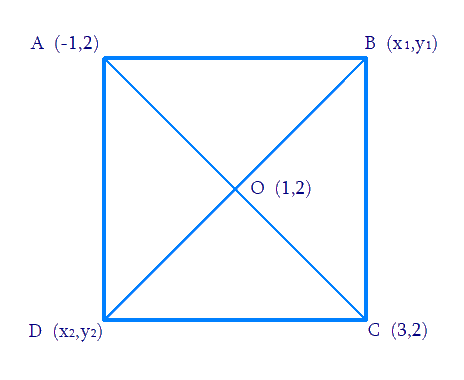# Ex.7.4 Q4 Coordinate Geometry Solution - NCERT Maths Class 10

Go back to  'Ex.7.4'

## Question

The two opposite vertices of a square are \begin{align}\left( { - 1,\,2} \right){\text{ }}{\text{ and}}\left( {3,\,2} \right).\end{align} Find the coordinates of the other two vertices.

Video Solution
Coordinate Geometry
Ex 7.4 | Question 4

## Text Solution

Reasoning:

What is known?

The $$x$$ and $$y$$ co-ordinates of the two opposite vertices of a square.

What is unknown?

The coordinates of the other two vertices.

Steps:

From the Figure,Given,

• Let $$ABCD$$ be a square having known vertices \begin{align}{A}\,\,\left( { - 1,{\text{ }}2} \right){\text{ and}}{\text{ }}{C}\,\,\left( {3,{\text{ }}2} \right)\end{align}as vertices $$A$$ and $$C$$ respectively.
• Let \begin{align}\text{B}\left( {x, y} \right)\end{align} be one unknown vertex

We know that the sides of a square are equal to each other.

\begin{align}\therefore {{AB = BC}}\end{align}

By Using Distance formula to find distance between points $$AB\; {\rm and } \;BC ,$$

\begin{align} \sqrt {{{(x_1 \!-\! 1)}^2} \!+\! {{(y_1 \!-\! 2)}^2}} &\!=\! \sqrt {{{(x_1 \!-\! 3)}^2} \!+\! {{(y_1 \!-\! 2)}^2}} \\ \begin{bmatrix}{x_1^2} \!+\! 2x \!+\! 1 \!+\\ {y_1^2} \!-\! 4y \!+\! 4\end{bmatrix} &\!=\! \begin{bmatrix}{x_1^2} \!+\! 9 \!-\! 6x \!+\! \\{y_1^2} \!+\! 4 \!-\! 4y _1\end{bmatrix}\\\text{(By Simplifying }&\text{Transposing)}\\\\8x_1 &\!=\! 8 \\ x_1 &\!=\! 1 \end{align}

We know that in a square, all interior angles are of \begin{align}{90^\circ }.\end{align}

In \begin{align}\Delta ABC\end{align}

\begin{align}{AB}^{2}+{BC}^{2}={AC}^{2} \\\\\text { [By Pythagoras theorem }] \end {align}

Distance formula is used to find distance between $$AB$$, $$BC$$ and $$AC$$

\begin{align} &{\left( {\sqrt {{{(1 + 1)}^2} + {{(y_1 - 2)}^2}} } \right)^2} + {\left( {\sqrt {{{(1 - 3)}^2} + {{(y_1 - 2)}^2}} } \right)^2}\\ &\qquad= {\left( {\sqrt {{{(3 + 1)}^2} + {{(2 - 2)}^2}} } \right)^2} \end{align}

\begin{align}\begin{bmatrix}4 + {y_1^2} + 4 - 4y_1 + \\4 + {y_1^2} - 4y_1 + 4\end{bmatrix}&= 16 \\2{y_1^2} + 16 - 8y &= 16 \\2{y_1^2} - 8y &= 0\\y_1(y_1 - 4) &= 0\\y_1 &= 0\,\,or\,\,4\end{align}

Hence the required vertices are \begin{align}B{\text{ }}\left( {1,\,\,0} \right){\text{ }}{\text{ and}}\;D{\text{ }}\left( {1,{\text{ }}4} \right)\end{align}

Learn from the best math teachers and top your exams

• Live one on one classroom and doubt clearing
• Practice worksheets in and after class for conceptual clarity
• Personalized curriculum to keep up with school